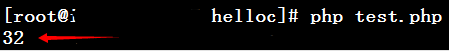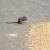# 如何编写 PHP 扩展 之 我的第一个PHP扩展

Cleey 发布于 2015/08/11 15:24

• 编写skel 原型
• 生成PHP扩展框架
• 编写自己的代码逻辑
• 编译成PHP扩展 *.so
• 拷贝到PHP扩展目录，重启PHP服务
• 调用自己的PHP扩展

` vim ./php-5.6.7/README.EXT_SKEL`

`<?php helloc(1022);?> // 输出为 32;`

`# cd  ./php-5.6.7/ext/ # vim  helloc.skel int helloc(int a int b)`

`# ./ext_skel --extname=helloc --proto=helloc.skel  `

`# cd helloc # vim config.m4`

```dnl PHP_ARG_WITH(helloc for helloc support
dnl Make sure that the comment is aligned: dnl [ --with-helloc Include helloc support])```

`PHP_ARG_WITH(helloc for helloc support Make sure that the comment is aligned: [ --with-helloc Include helloc support]) `

`# vim helloc.c`

`PHP_FUNCTION(helloc) { int argc = ZEND_NUM_ARGS(); long a; long b; long re; // 新增的 if (zend_parse_parameters(argc TSRMLS_CC "ll" &a &b) == FAILURE) return; re = a+b; // 新增的 RETURN_LONG(re); // 新增的 }`

`# /usr/bin/phpize  # ./configure --with-php-config=/usr/bin/php-config # make`

`# cp modules/helloc.so /usr/lib64/php/modules/ # vim /etc/php.ini           // 为php配置文件加上扩展（文件末尾就行） extension=helloc.so # service php-fpm restart    // 这里你用的apache那么就需要重启 apache  `

`# vim test.php <?php echo helloc(1022); ?> # php test.php`0```zval *arr;
char *primary_key;
long primary_key_len;
char *parent_key;
long parent_key_len;
char *nodes_name;
long nodes_name_len;
//long index;
HashTable *arr_hash;
PHP_FUNCTION(arraytree)
{
long index;

if (zend_parse_parameters(ZEND_NUM_ARGS() TSRMLS_CC,"asssl",&arr,&primary_key,&primary_key_len,&parent_key,&parent_key_len,&nodes_name,&nodes_name_len,&index) == FAILURE){
RETURN_NULL();
}

arr_hash = Z_ARRVAL_P(arr);
array_init(return_value);
HashPosition pos;
zval **z_item;
for(zend_hash_internal_pointer_reset_ex(arr_hash,&pos); zend_hash_get_current_data_ex(arr_hash,(void**) &z_item,&pos) == SUCCESS; zend_hash_move_forward_ex(arr_hash,&pos)){
if(Z_TYPE_PP(z_item) == IS_ARRAY){
HashTable *arr_hash_1 = Z_ARRVAL_PP(z_item);
zval **parent_key_val;
if(zend_hash_exists(arr_hash_1,parent_key,parent_key_len) == SUCCESS){
if(zend_hash_find(arr_hash_1,parent_key,parent_key_len + 1,(void **) &parent_key_val) == SUCCESS){
convert_to_long_ex(parent_key_val);
if(Z_LVAL_PP(parent_key_val) == index){
zval *tem_z;
tem_z = *z_item;
if(zend_hash_exists(arr_hash_1,primary_key,primary_key_len) == SUCCESS){
zval **primary_key_val;
if(zend_hash_find(arr_hash_1,primary_key,primary_key_len + 1,(void **) &primary_key_val) == SUCCESS){
convert_to_long_ex(primary_key_val);
zval *nodes_arr = get_tree(Z_LVAL_PP(primary_key_val));
//								_zend_hash_index_update_or_next_insert();
}
}
}
}
}
}
}
}
zval* get_tree(long i){
HashPosition pos;
zval **z_item;
//ALLOC_INIT_ZVAL(arr);
//array_init(arr);//zval *arr;
zval *arr;
MAKE_STD_ZVAL(arr);
array_init(arr);
for(zend_hash_internal_pointer_reset_ex(arr_hash,&pos); zend_hash_get_current_data_ex(arr_hash,(void**) &z_item,&pos) == SUCCESS; zend_hash_move_forward_ex(arr_hash,&pos)){
if(Z_TYPE_PP(z_item) == IS_ARRAY){
HashTable *arr_hash_1 = Z_ARRVAL_PP(z_item);
zval **parent_key_val;
if(zend_hash_exists(arr_hash_1,parent_key,parent_key_len) == SUCCESS){
if(zend_hash_find(arr_hash_1,parent_key,parent_key_len + 1,(void **) &parent_key_val) == SUCCESS){
convert_to_long_ex(parent_key_val);
if(Z_LVAL_PP(parent_key_val) == i){
zval *tem_z;
tem_z = *z_item;
if(zend_hash_exists(arr_hash_1,primary_key,primary_key_len) == SUCCESS){
zval **primary_key_val;
if(zend_hash_find(arr_hash_1,primary_key,primary_key_len + 1,(void **) &primary_key_val) == SUCCESS){
convert_to_long_ex(primary_key_val);
zval *nodes_arr = get_tree(Z_LVAL_PP(primary_key_val));
//php_printf("[%s]",Z_TYPE_P(nodes_arr));
//zend_hash_update(tem_z->value.ht,nodes_name,nodes_name_len+1,(void *)&nodes_arr,sizeof(zval *), NULL);
}
}
}
}
}
}
}
return arr;
}```

00